Examples

Practical Geometry
Serial order wise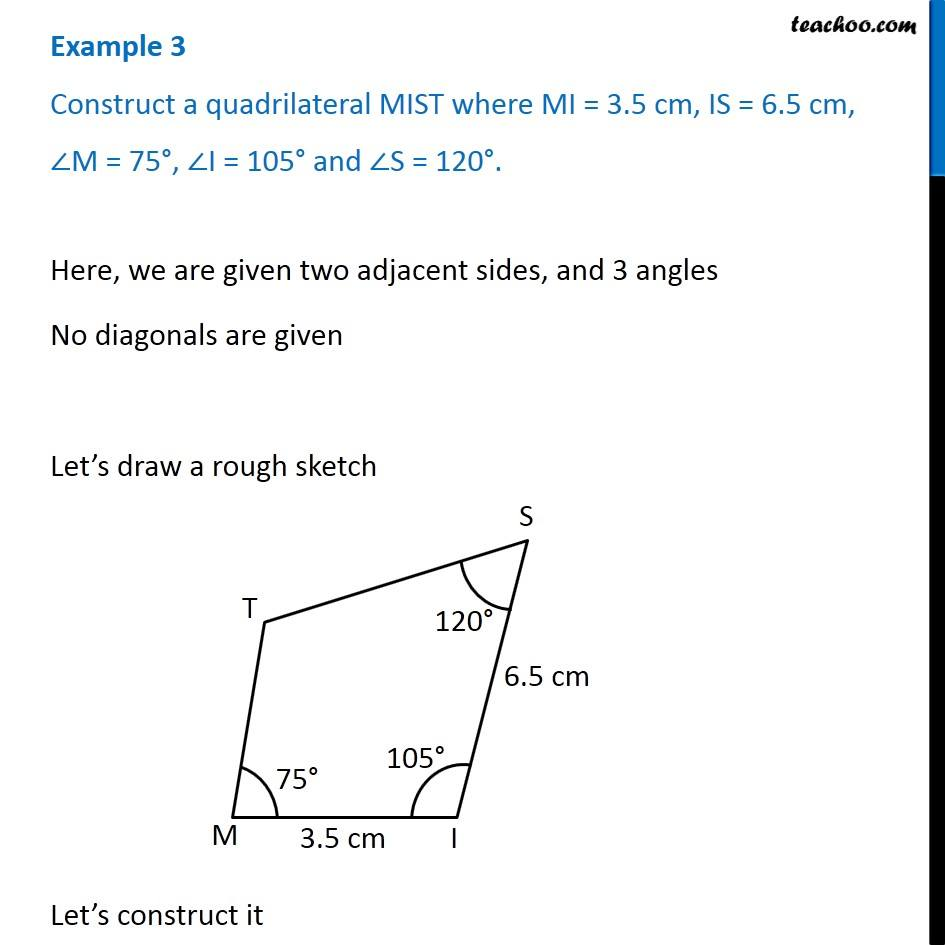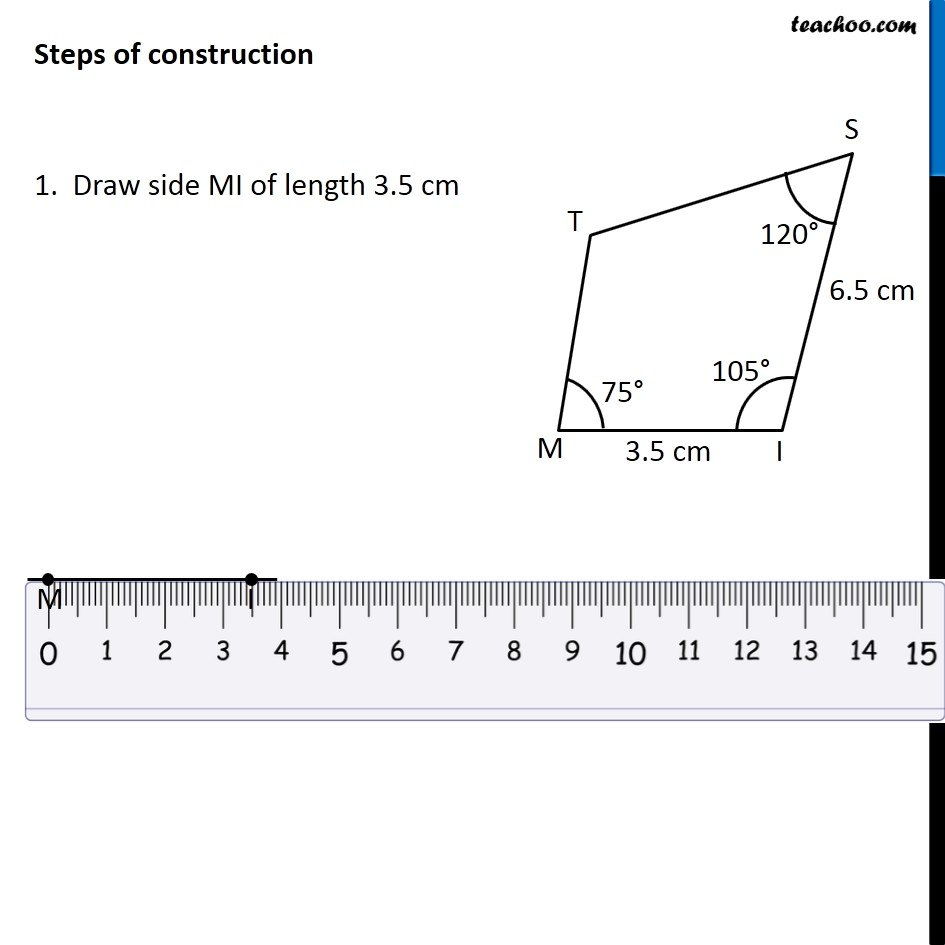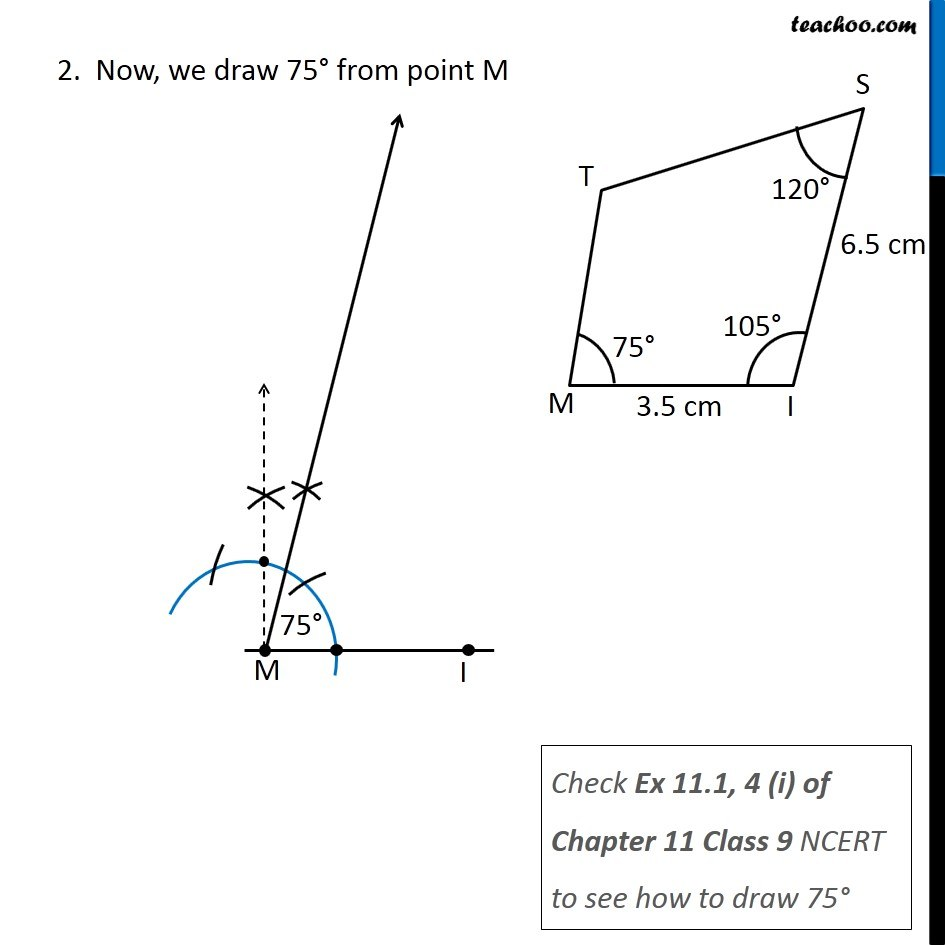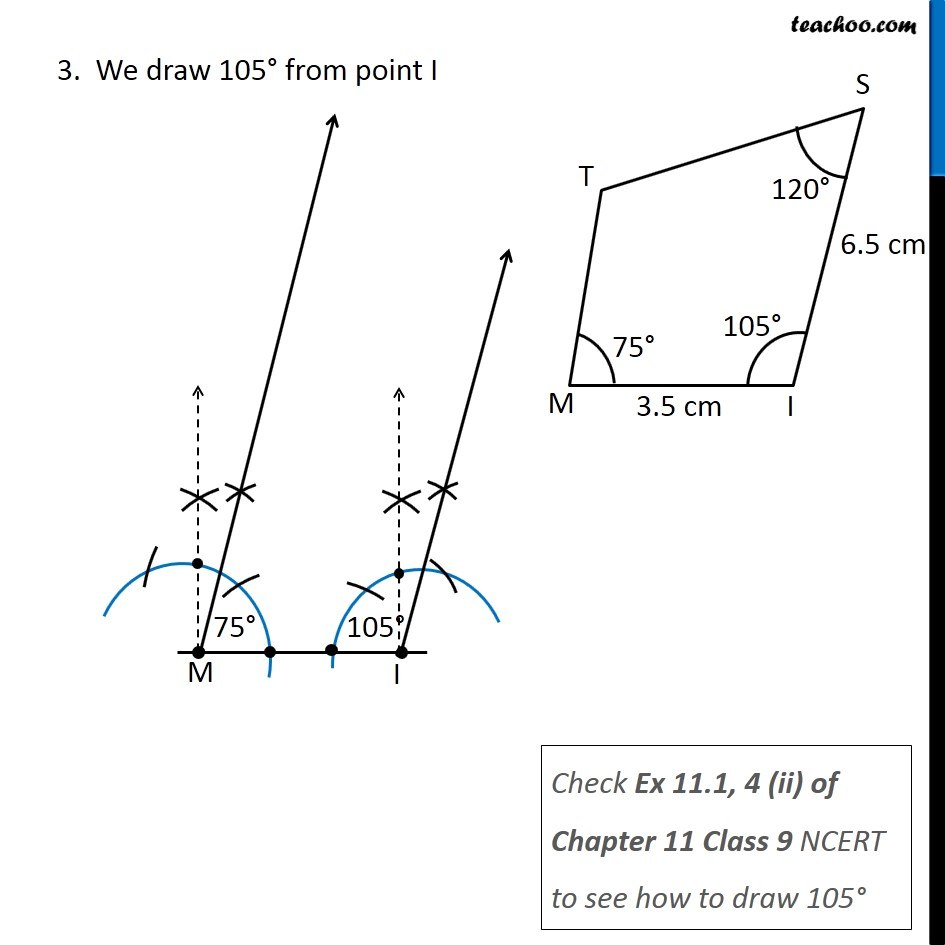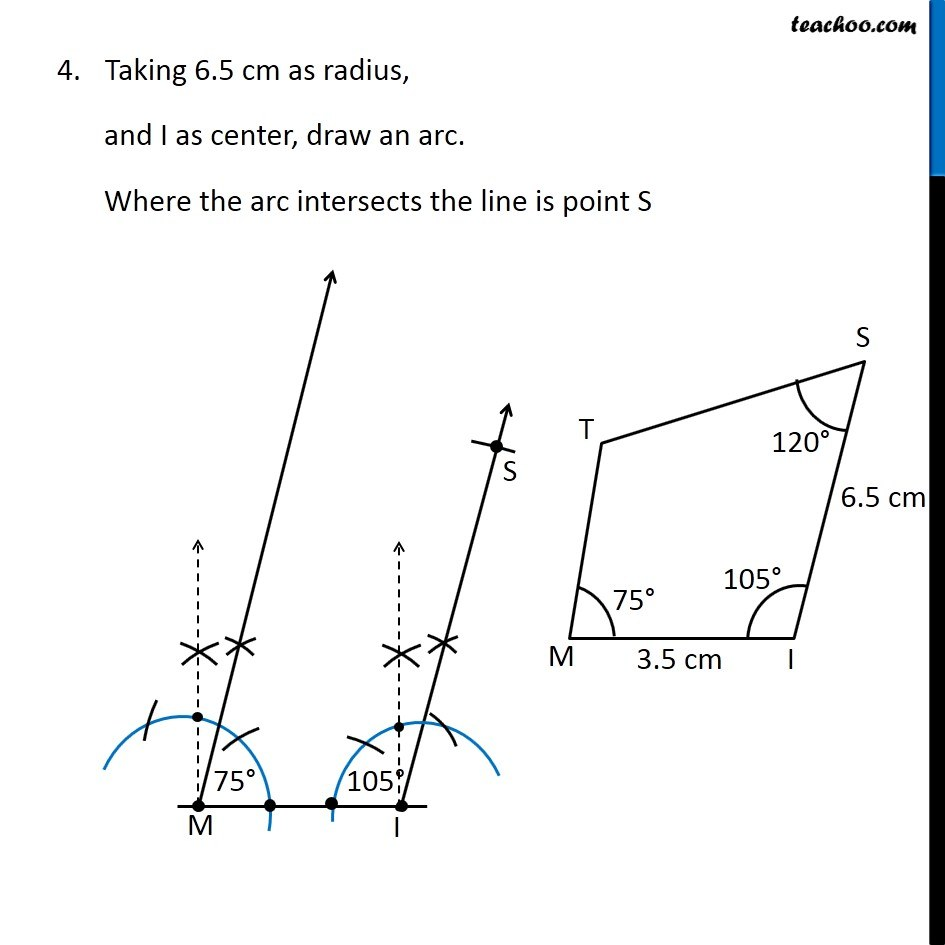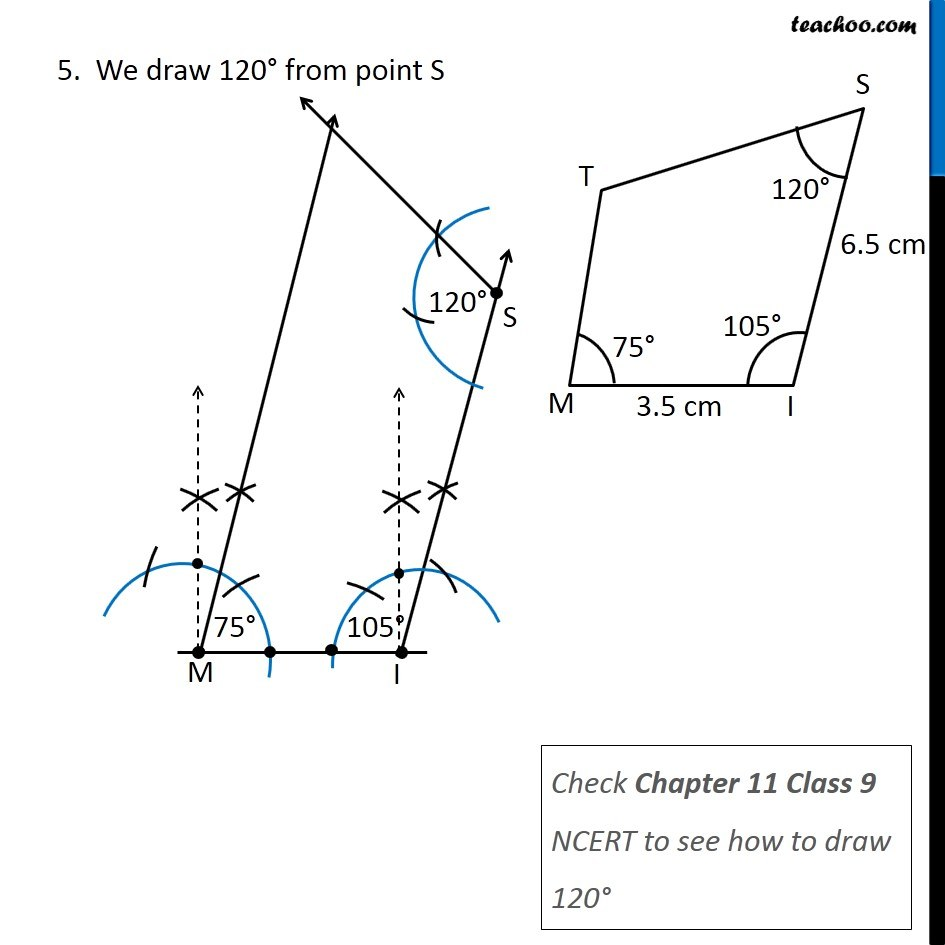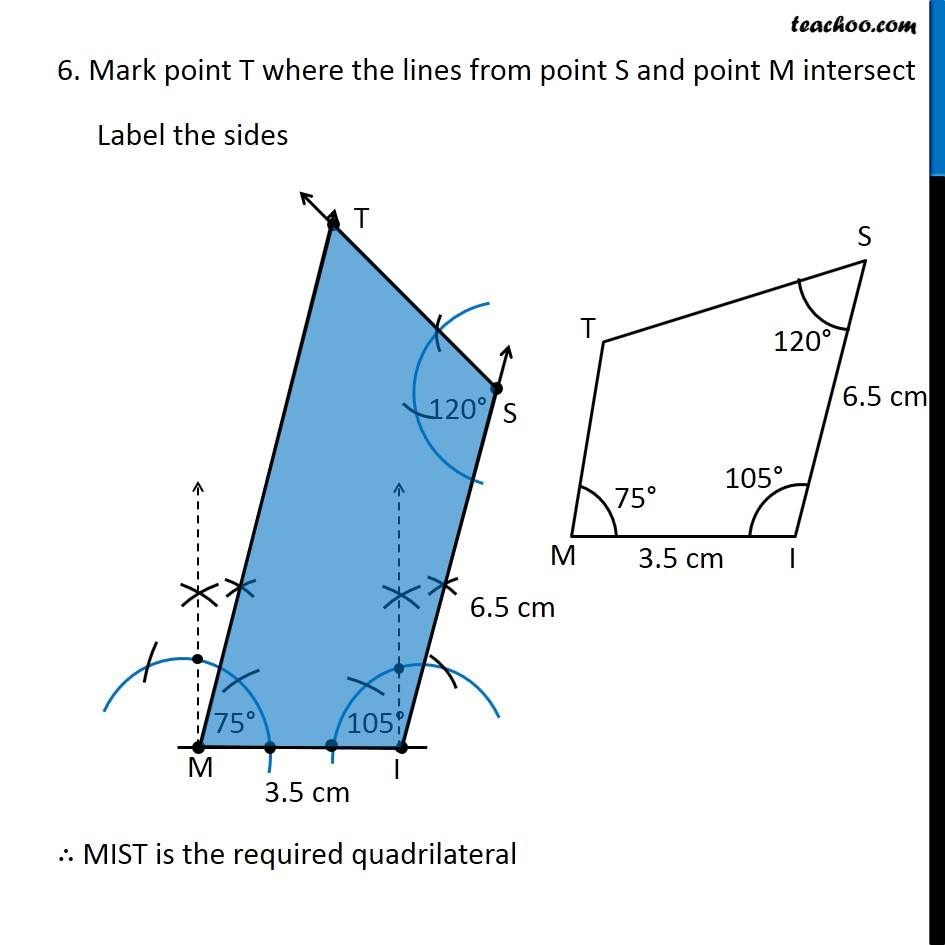Learn in your speed, with individual attention - Teachoo Maths 1-on-1 Class

### Transcript

Example 3 Construct a quadrilateral MIST where MI = 3.5 cm, IS = 6.5 cm, ∠M = 75°, ∠I = 105° and ∠S = 120°. Here, we are given two adjacent sides, and 3 angles No diagonals are given Let’s draw a rough sketch Let’s construct it Here, we are given two adjacent sides, and 3 angles No diagonals are given Let’s draw a rough sketch Let’s construct it 2. Now, we draw 75° from point M Check Ex 11.1, 4 (i) of Chapter 11 Class 9 NCERT to see how to draw 75° 3. We draw 105° from point I Check Ex 11.1, 4 (ii) of Chapter 11 Class 9 NCERT to see how to draw 105° Taking 6.5 cm as radius, and I as center, draw an arc. Where the arc intersects the line is point S 5. We draw 120° from point S Check Chapter 11 Class 9 NCERT to see how to draw 120° 6. Mark point T where the lines from point S and point M intersect Label the sides ∴ MIST is the required quadrilateral